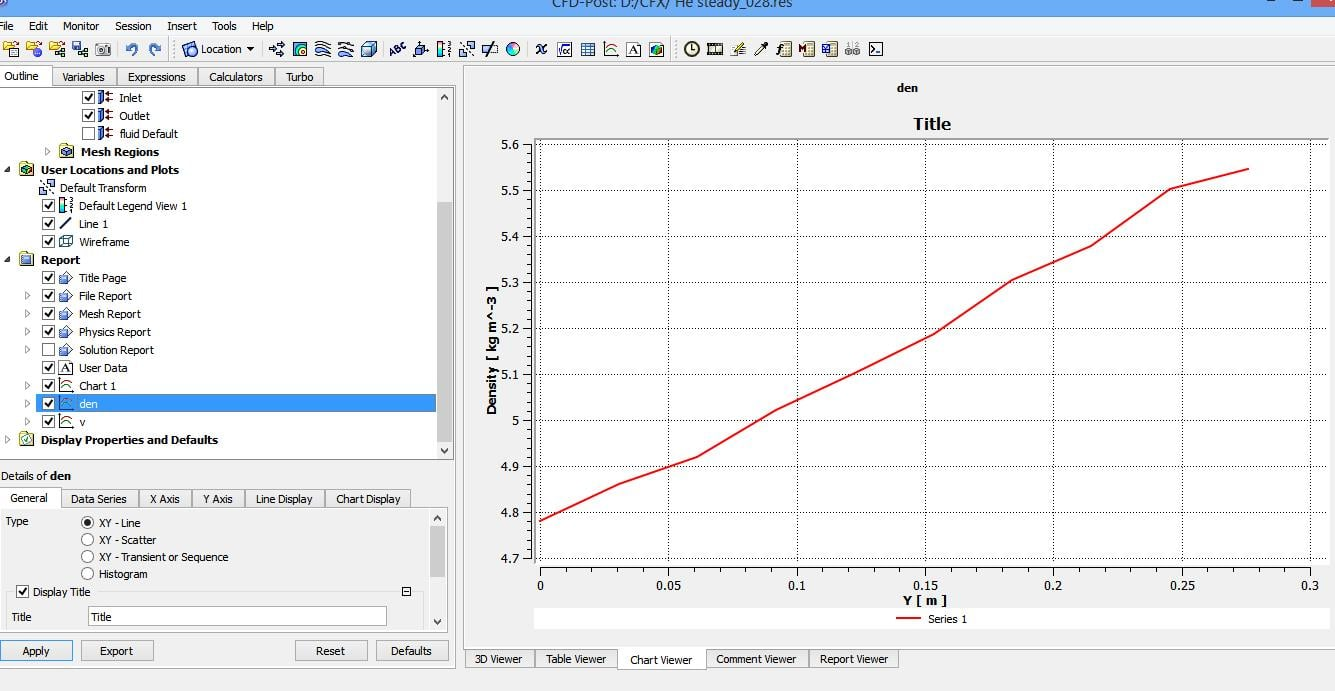## Fluids

•hamda
Subscriber

Hello,

I am working on a problem in which mass flow rate is constant. I specified mass flow rate in "inlet" boundary condition. But after seeing the results (velocity and density) I realized that the mass flow rate wasn't constant. It should be mentioned the fluid is helium and it is considered as an ideal gas. Now I was wondering how I can define mass flow rate as a constant value into pre CFX or if I can't do that, How can I define velocity or density as a function of each other to keep mass flow rate constant.

•Rob
Ansys Employee

Check the flux on the boundary: if the model is converged it'll be as you set. I suspect you've not accounted for something when doing the velocity & density maths.

•hamda
Subscriber

I think maybe the mass flow rate area  (A) is not constant in my geometry. As you see  fluid should pass through these spheres but  A would be the same at inlet and outlet  but the value of (density *velocity) is not the same for both. in Boundary conditions : I selected "as specified" for mass flow rate area  and then I changed into "total for all all sectors" but I didn't see any difference.•Rob
Ansys Employee

I assume you mean it flows between the spheres? Monitor the inlet mass flow & outlet mass flow: are they equal and are they what you expect?